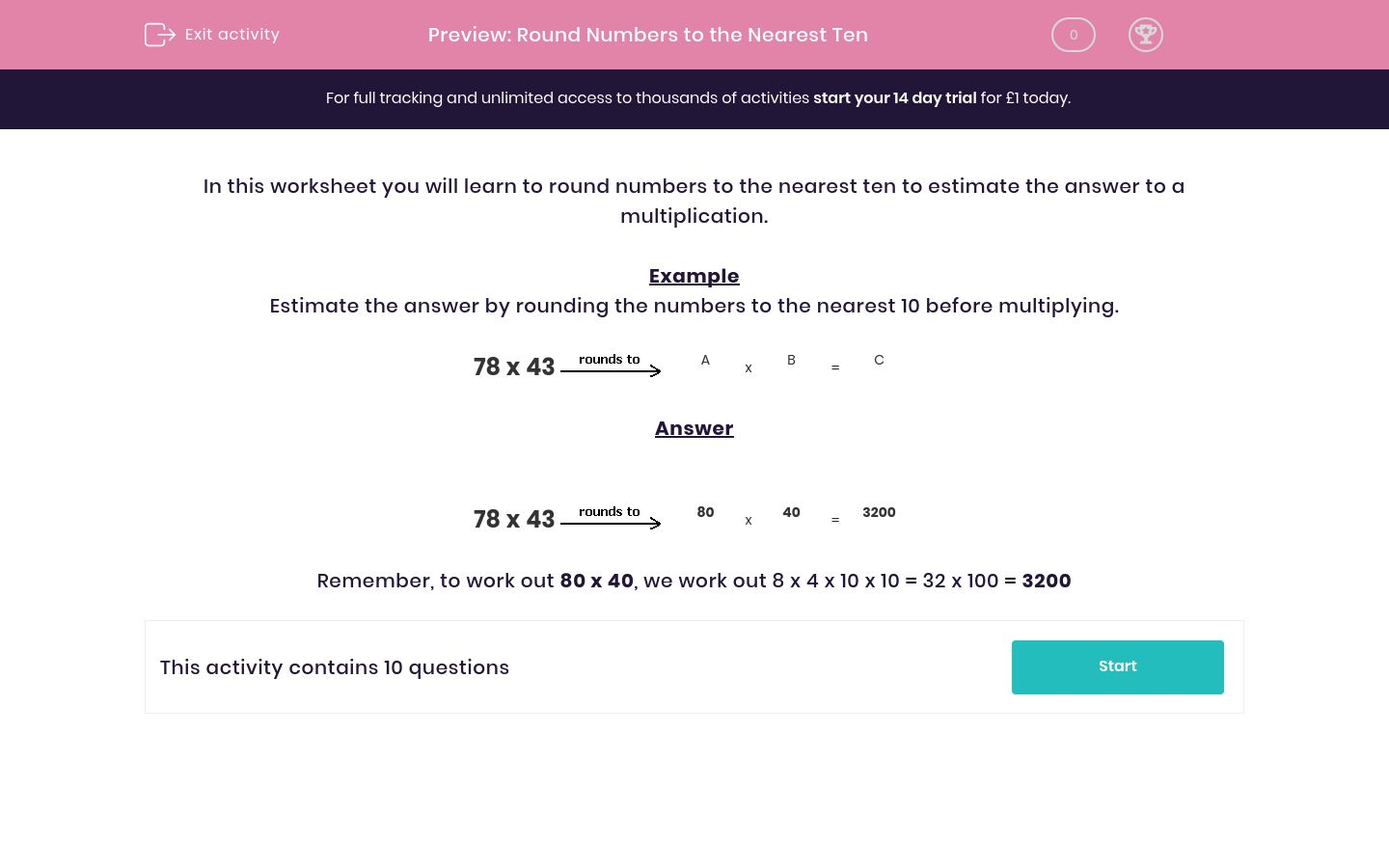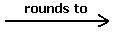# Round Numbers to the Nearest Ten

In this worksheet, students round numbers to the nearest 10 before multiplying to find an estimated answer.Key stage:  KS 2

Curriculum topic:   Maths and Numerical Reasoning

Curriculum subtopic:   Place Value

Difficulty level:### QUESTION 1 of 10

In this worksheet you will learn to round numbers to the nearest ten to estimate the answer to a multiplication.

Example

Estimate the answer by rounding the numbers to the nearest 10 before multiplying.

78 x 43A
x
 B
=
 C

78 x 4380
x
 40
=
 3200

Remember, to work out 80 x 40, we work out 8 x 4 x 10 x 10 = 32 x 100 = 3200

Estimate the answer by rounding the numbers to the nearest 10 before multiplying to find C.

67 x 32A
x
 B
=
 C

## Column B

A
30
B
70
C
2100

Estimate the answer by rounding the numbers to the nearest 10 before multiplying to find C.

59 x 32A
x
 B
=
 C

## Column B

A
30
B
1800
C
60

Estimate the answer by rounding the numbers to the nearest 10 before multiplying to find C.

50 x 28A
x
 B
=
 C

## Column B

A
30
B
1500
C
50

Estimate the answer by rounding the numbers to the nearest 10 before multiplying to find C.

37 x 20A
x
 B
=
 C

## Column B

A
800
B
40
C
20

Estimate the answer by rounding the numbers to the nearest 10 before multiplying to find C.

73 x 30A
x
 B
=
 C

## Column B

A
70
B
2100
C
30

Estimate the answer by rounding the numbers to the nearest 10 before multiplying to find C.

31 x 28A
x
 B
=
 C

## Column B

A
30
B
900
C
30

Estimate the answer by rounding the numbers to the nearest 10 before multiplying to find C.

43 x 19A
x
 B
=
 C

## Column B

A
20
B
800
C
40

Estimate the answer by rounding the numbers to the nearest 10 before multiplying to find C.

36 x 29A
x
 B
=
 C

## Column B

A
30
B
1200
C
40

Estimate the answer by rounding the numbers to the nearest 10 before multiplying to find C.

97 x 83A
x
 B
=
 C

## Column B

A
8000
B
80
C
100

Estimate the answer by rounding the numbers to the nearest 10 before multiplying to find C.

27 x 12A
x
 B
=
 C

## Column B

A
10
B
300
C
30
• Question 1

Estimate the answer by rounding the numbers to the nearest 10 before multiplying to find C.

67 x 32A
x
 B
=
 C

## Column B

A
70
B
30
C
2100
• Question 2

Estimate the answer by rounding the numbers to the nearest 10 before multiplying to find C.

59 x 32A
x
 B
=
 C

## Column B

A
60
B
30
C
1800
• Question 3

Estimate the answer by rounding the numbers to the nearest 10 before multiplying to find C.

50 x 28A
x
 B
=
 C

## Column B

A
50
B
30
C
1500
• Question 4

Estimate the answer by rounding the numbers to the nearest 10 before multiplying to find C.

37 x 20A
x
 B
=
 C

## Column B

A
40
B
20
C
800
• Question 5

Estimate the answer by rounding the numbers to the nearest 10 before multiplying to find C.

73 x 30A
x
 B
=
 C

## Column B

A
70
B
30
C
2100
• Question 6

Estimate the answer by rounding the numbers to the nearest 10 before multiplying to find C.

31 x 28A
x
 B
=
 C

## Column B

A
30
B
30
C
900
• Question 7

Estimate the answer by rounding the numbers to the nearest 10 before multiplying to find C.

43 x 19A
x
 B
=
 C

## Column B

A
40
B
20
C
800
• Question 8

Estimate the answer by rounding the numbers to the nearest 10 before multiplying to find C.

36 x 29A
x
 B
=
 C

## Column B

A
40
B
30
C
1200
• Question 9

Estimate the answer by rounding the numbers to the nearest 10 before multiplying to find C.

97 x 83A
x
 B
=
 C

## Column B

A
100
B
80
C
8000
• Question 10

Estimate the answer by rounding the numbers to the nearest 10 before multiplying to find C.

27 x 12A
x
 B
=
 C

## Column B

A
30
B
10
C
300
---- OR ----

Sign up for a £1 trial so you can track and measure your child's progress on this activity.

### What is EdPlace?

We're your National Curriculum aligned online education content provider helping each child succeed in English, maths and science from year 1 to GCSE. With an EdPlace account you’ll be able to track and measure progress, helping each child achieve their best. We build confidence and attainment by personalising each child’s learning at a level that suits them.

Get started